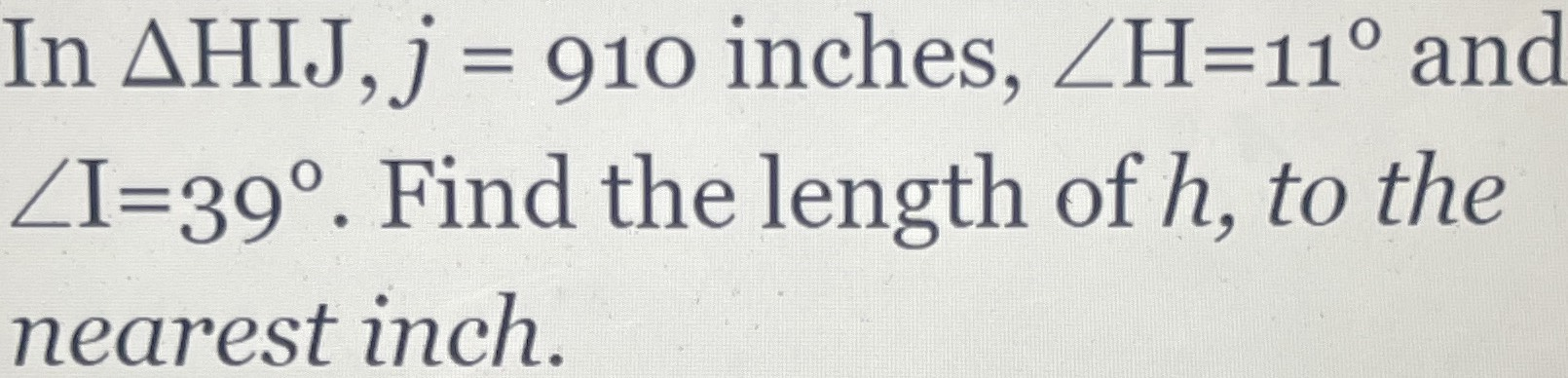### Still have math questions?

Trigonometry
QuestionIn $$\Delta HIJ , j = 910$$ inches, $$\angle H = 11 ^ { \circ }$$ and

$$\angle I = 39 ^ { \circ } .$$ Find the length of $$h$$ , to the nearest inch.